# 监控摄像头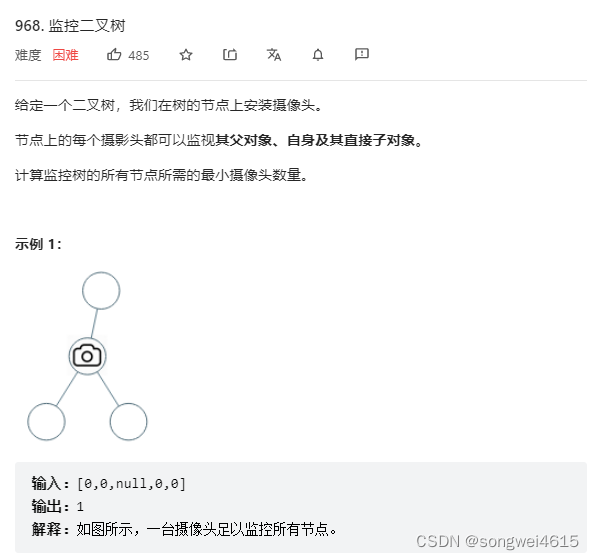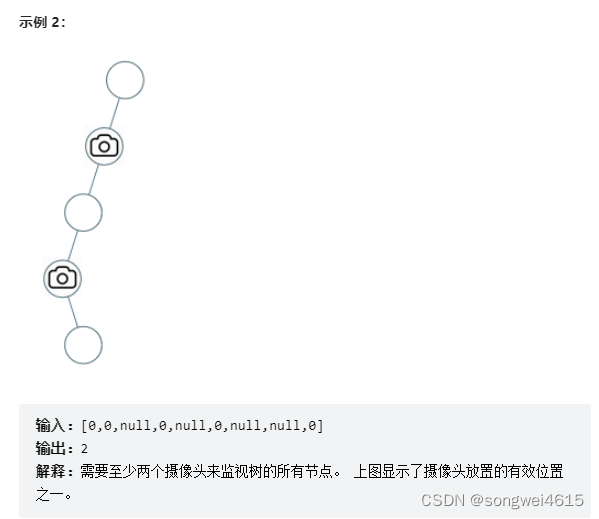0：该节点无覆盖
1：本节点有摄像头
2：本节点有覆盖

• 情况1：左右节点都有覆盖
左孩子有覆盖，右孩子有覆盖，那么此时中间节点应该就是无覆盖的状态了。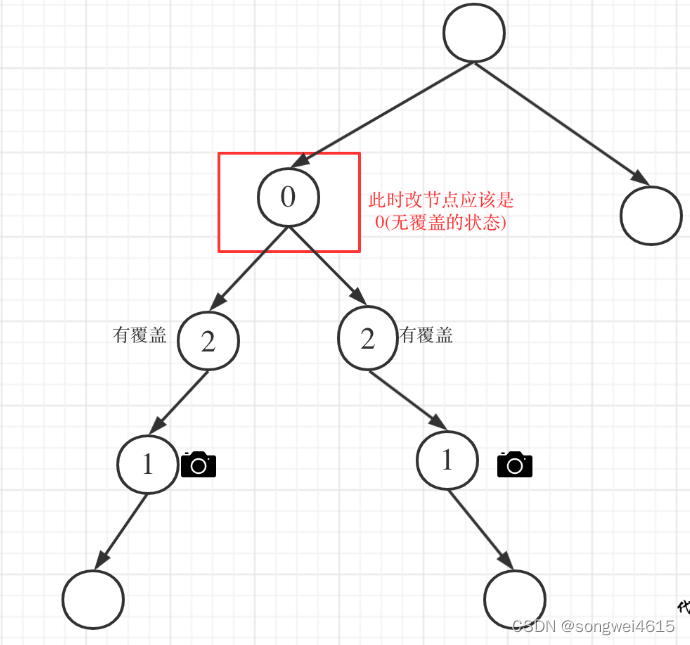• 情况2：左右节点至少有一个无覆盖的情况
如果是以下情况，则中间节点（父节点）应该放摄像头：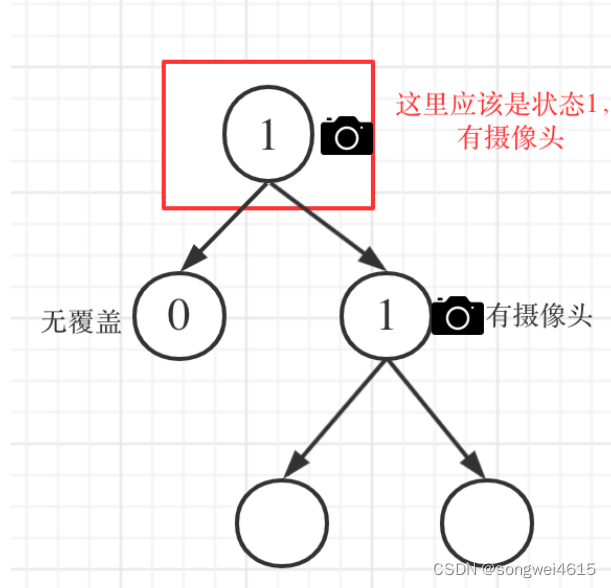• 情况3：左右节点至少有一个有摄像头
如果是以下情况，其实就是 左右孩子节点有一个有摄像头了，那么其父节点就应该是2（覆盖的状态）
• 情况4：头结点没有覆盖
以上都处理完了，递归结束之后，可能头结点 还有一个无覆盖的情况，如图：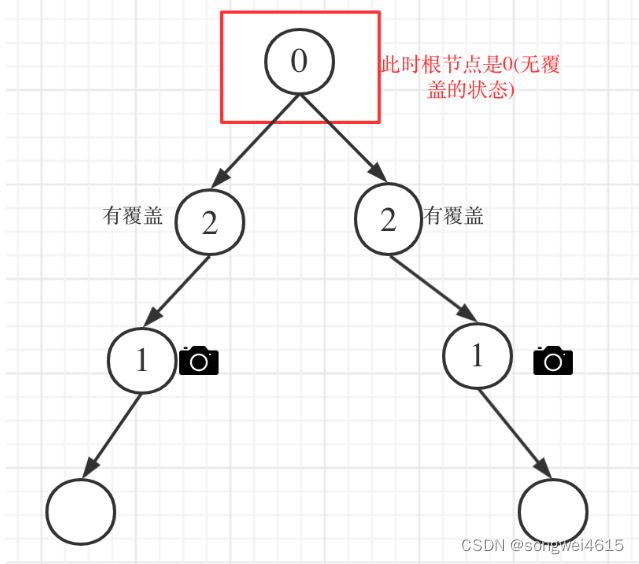``````class Solution {
private:int result;int traversal(TreeNode* cur) {// 空节点，该节点有覆盖if (cur == NULL) return 2;int left = traversal(cur->left);    // 左int right = traversal(cur->right);  // 右// 情况1// 左右节点都有覆盖if (left == 2 && right == 2) return 0;// 情况2// left == 0 && right == 0 左右节点无覆盖// left == 1 && right == 0 左节点有摄像头，右节点无覆盖// left == 0 && right == 1 左节点有无覆盖，右节点摄像头// left == 0 && right == 2 左节点无覆盖，右节点覆盖// left == 2 && right == 0 左节点覆盖，右节点无覆盖if (left == 0 || right == 0) {result++;return 1;}// 情况3// left == 1 && right == 2 左节点有摄像头，右节点有覆盖// left == 2 && right == 1 左节点有覆盖，右节点有摄像头// left == 1 && right == 1 左右节点都有摄像头// 其他情况前段代码均已覆盖if (left == 1 || right == 1) return 2;// 以上代码我没有使用else，主要是为了把各个分支条件展现出来，这样代码有助于读者理解// 这个 return -1 逻辑不会走到这里。return -1;}public:int minCameraCover(TreeNode* root) {result = 0;// 情况4if (traversal(root) == 0) { // root 无覆盖result++;}return result;}
};
``````

## 二刷

``````/*** Definition for a binary tree node.* struct TreeNode {*     int val;*     TreeNode *left;*     TreeNode *right;*     TreeNode() : val(0), left(nullptr), right(nullptr) {}*     TreeNode(int x) : val(x), left(nullptr), right(nullptr) {}*     TreeNode(int x, TreeNode *left, TreeNode *right) : val(x), left(left), right(right) {}* };*/
class Solution {
public:int result = 0;int track_back(TreeNode* cur){if(cur==nullptr) return 2;//有覆盖int left = track_back(cur->left);int right = track_back(cur->right);if(left==2&&right==2) return 0;//无覆盖if(left==0||right==0) {result++;return 1;//放摄像头}if(left==1||right==1) return 2;return -1;}int minCameraCover(TreeNode* root) {if(track_back(root)==0) result++;return result;}
};
``````